##### Statistics: 1001 Practice Problems For Dummies (+ Free Online Practice)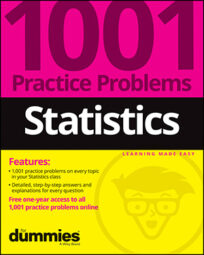The problems presented here give you practice calculating and interpreting the mean, variance, and standard deviation of a random variable. In the following table, X represents the number of siblings for the 29 students in a first-grade class.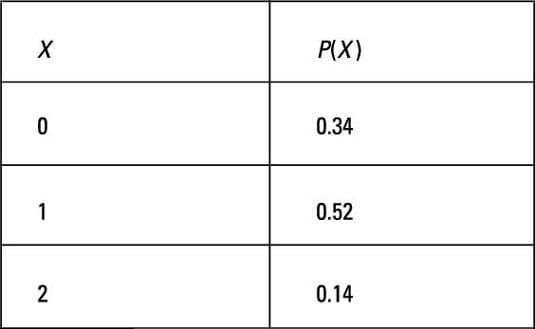## Sample questions

1. What is the mean number of siblings for these students?

In this case, X represents the number of siblings a student has. The question asks for the mean of X, designated as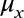The possible values of X are 0, 1, and 2, denoted x1, x2, and x3, respectively; their proportions (probabilities) are equal to 0.34, 0.52, and 0.14 (denoted p1, p2, and p3, respectively).

To find the mean of X, multiply each value, xi, by its probability, pi, and then add the products: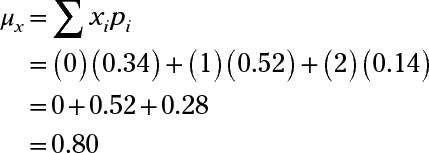2. What is the variance for the number of siblings for these students?

To find the variance of X, you take the first value of X, call it x1, subtract the mean of X, square that result, and then multiply it by the probability for x1 (denoted p1). Do the same thing for every other possible value of X, and then sum up all the results.

In this case, X represents the number of siblings. The values of X are 0, 1, and 2, denoted x1, x2, and x3, respectively. Their probabilities are 0.34, 0.52, and 0.14, respectively.

You need to first find the mean of X because it’s part of the formula to calculate the variance. Multiply each value, xi, by its probability, pi, and then add the products:Now, plug this value into the formula for finding the variance: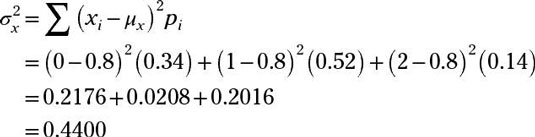3. What is the standard deviation for the number of siblings for these students? Round your answer to two decimal places.

To find the standard deviation of X, you first find the variance of X, and then take the square root of that result.

To find the variance of X, you take the first value of X, call it x1, subtract the mean of X, and square the result. Then, you multiply that result by the probability for x1, denoted p1. Do this for each possible value of X, and then add up all the results.

In this case, X represents the number of siblings. The values of X are 0, 1, and 2, denoted x1, x2, and x3, respectively. Their probabilities are 0.34, 0.52, and 0.14, respectively.

You need to first find the mean of X because it’s part of the formula to calculate the variance. Multiply each value, xi, by its probability, pi, and then add the products:Now, plug this value into the formula to calculate the variance of X:Finally, find the standard deviation of X. The standard deviation is the square root of the variance, or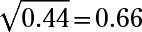If you need more practice on this and other topics from your statistics course, visit 1,001 Statistics Practice Problems For Dummies to purchase online access to 1,001 statistics practice problems! We can help you track your performance, see where you need to study, and create customized problem sets to master your stats skills.